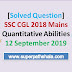## SSC CGL 2018 Mains Quantitative Abilities Solution 12 September 2019

Dear Students, SSC CGL 2018 Mains Question Paper and Answer Key have released. Here you will get solution of Maths paper of CGL Mains. 1-10 Quantitative aptitude questions are solved here next 10 questions will be solved in another post. If you found any mistake in question or solution report it at comment section. Here each question solved with short trick so if you have any doubt about a question post your query in comment section. SSC CGL 2018 mains maths solved question paper 12 September 2019 given below:

Q.1 If the curved surface area of a solid cylinder is one-third of its total surface area, then what is the ratio of its diameter to its height?
1. 4:1
2. 5:2
3. 1:1
4. 2:1

Q.2 A sum lent out at simple interest amounts to 6076 in 1 year and 7504 in 4 years. The sum and the rate of interest p.a. are respectively:
1. ₹55,600 and 9%
2. ₹5,600 and 8.5%
3. ₹35,400 and 9%
4. ₹55,400 and 10%

Q.3 In a quadrilateral ABCD, the bisectors of ∠C and ∠D meet at E. If ∠CED= 56° and ∠A= 49°, then the measure of ∠B is:
1. 54°
2. 63°
3. 71°
4. 67°

Q.4 If $3 (cot^2Ø - cos^2Ø) = cos^2Ø$, 0°<Ø< 90°, then the value of $(tan^2Ø+ cosec^2Ø+ sin^2Ø)$ is:
1. 25/12
2. 61/12
3. 15/4
4. 13/3

Q.5  The value of $\displaystyle\frac{(0.545) (0.081)(0.51)(5.2)}{(0.324)^3 +(0.221)^3-(0.545)^3}$
1. -1
2. -3
3. 1
4. 3

Q.6 What is the ratio of the third proportional to 0.4 and 0.8, to the mean proportional between 13.5 and 0.24?
1. 5:4
2. 9:10
3. 7:8
4. 8:9

Q.7 The value of $\displaystyle9 × 6 ÷ 24 + 8 ÷ 2 of 5 - 30 ÷ 4 of 4 + 27 × 5 ÷ 9$ is:
1. 647/40
2. 259/8
3. 243/8
4. 493/8

Q.8 The value of $\displaystyle\frac{sin(78°+θ)-cos(12-θ)+(tan^270°-cosec^220°)}{sin25°cos65°+cos25°sin65°}$ is:
1. 0
2. -2
3. 2
4. -1

Q.9 The value of $\displaystyle(1\frac{1}{3} ÷ 2\frac{6}{7} of 5\frac{3}{5}) ÷ (6\frac{2}{3} ÷ 4\frac{1}{2} of 5\frac{1}{3}) × (\frac{3}{4} × 2\frac{2}{3} ÷ \frac{5}{9} of 1\frac{1}{5}) = 1 + k$, where k lies between:
1. -0.06 and -0.05
2. -0.05 and - 0.04
3. -0.07 and - 0.06
4. -0.08 and - 0.07

Q.10 In ΔABC, AB = AC and D is a point on BC. If BD = 5 cm, AB = 12 cm and AD = 8 cm, then the length of CD is:
1. 14.8 cm
2. 16.2 cm
3. 14 cm
4. 16 cm

Solutions

[Solution] SSC CGL 2018 Mains Quantitative Abilities 12 September 2019Reviewed by Super Pathshala on 13:50 Rating: 5